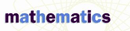# Accuracy and Precision - Research Article from Macmillan Science Library: Mathematics

This encyclopedia article consists of approximately 4 pages of information about Accuracy and Precision.
 This section contains 874 words (approx. 3 pages at 300 words per page) View a FREE sampleAccuracy and precision both concern the quality of a measure. However, the terms have different meanings and should not be used as substitutes for one another.

## Precision

Precision depends on the unit used to obtain a measure. The smaller the unit, the more precise the measure. Consider measures of time, such as 12 seconds and 12 days. A measurement of 12 seconds implies a time between11.5 and 12.5 seconds. This measurement is precise to the nearest second, with a maximum potential error of 0.5 seconds. A time of 12 days is far less precise. Twelve days suggests a time between 11.5 and 12.5 days, yielding a potential error of 0.5 days, or 43,200 seconds! Because the potential error is greater, the measure is less precise. Thus, as the length of the unit increases, the measure becomes less precise.

The number of decimal places in a measurement also affects precision. A time of 12.1 seconds is...

 This section contains 874 words (approx. 3 pages at 300 words per page) View a FREE sample